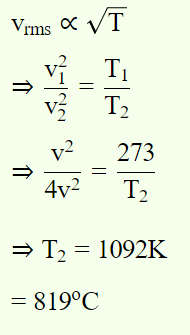## Kinetic Theory Questions and Answers Part-16

1. When temperature of an ideal gas is increased from $27^{\circ} C$  to $227^{\circ} C$ , its r.m.s. speed changed from 400 metre/sec to $V_{s}$ . The $V_{s}$ is
a) 516 metre/sec
b) 450 metre/sec
c) 310 metre/sec
d) 746 metre/sec

Explanation:2. The velocity of the molecules of a gas at temperature 120K is v . At what temperature will the velocity be 2v
a) 120 K
b) 240 K
c) 480 K
d) 1120 K

Explanation:3. If the pressure in a closed vessel is reduced by drawing out some gas, the mean free path of the molecules
a) Is decreased
b) Is increased
c) Remains unchanged
d) Increases or decreases according to the nature of the gas

Explanation: If the pressure in a closed vessel is reduced by drawing out some gas, the mean free path of the molecules is increased

4. At constant volume, temperature is increased. Then
a) Collision on walls will be less
b) Number of collisions per unit time will increase
c) Collisions will be in straight lines
d) Collisions will not change

Explanation: At constant volume, temperature is increased. Then, Number of collisions per unit time will increase

5. The r.m.s. speed of the molecules of a gas at a pressure $10^{5}$ Pa and temperature $0^{\circ} C$  is 0.5 $km sec^{-1}$  . If the pressure is kept constant but temperature is raised to 819°C, the velocity will become
a) $1.5kms^{-1}$
b) $2kms^{-1}$
c) $5kms^{-1}$
d) $1kms^{-1}$

Explanation: $1kms^{-1}$

6. At what temperature r.m.s. speed of air molecules doubles of that at N.T.P. is
a) 819°C
b) 719°C
c) 909°C
d) None of these

Explanation:7. The r.m.s. speed of a certain gas is v at 400K. The temperature at which the r.m.s. speed becomes two times, will be
a) 800 K
b) 1600 K
c) 1200 K
d) None of these

Explanation: 1600 K

8. The gas having average speed four times as that of $SO_{2}$ (molecular mass 64) is
a) He (molecular mass 4)
b) $O_{2}$ (molecular mass 32)
c) $H_{2}$ (molecular mass 2)
d) $CH_{4}$ (molecular mass 16)

Explanation: He (molecular mass 4)

9. A monoatomic gas molecule has
a) Three degrees of freedom
b) Four degrees of freedom
c) Five degrees of freedom
d) Six degrees of freedom